# How to calculate the volume of the log: best practices

The cubic capacity of a log is the volume of wood material, measured in cubic meters. This value is used in the construction of wooden log houses to calculate the quantity of roundwood. It is important to determine the cubic capacity when selling firewood, where payment is made for each cubic meter of wood. How to calculate the volume of round cylindrical lumber correctly?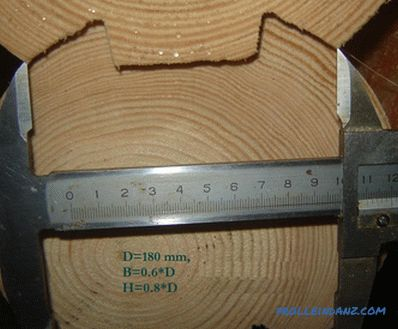You need to know the cubic capacity of the tree when buying this building material, since payment is made for each cubic meter.

There are several ways to estimate the volume of roundwood or firewood. All tried and tested techniques use data of measurements of geometric dimensions and mathematical calculations. Roulette is traditionally used to measure construction values.

## Calculation of cubic capacity of occupied space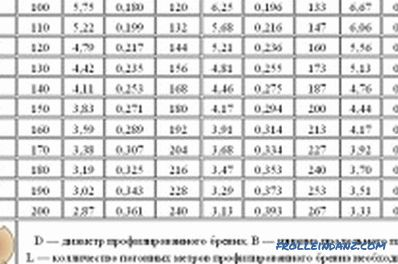Table of calculation of cubic capacity of logs

The easiest way is to define cubic rectangular space in which the timber is complex. Such a place can be a dump truck or a barn. You can also calculate the geometric dimensions of the woodpile, if it is folded in the form of a neat rectangle.

Since the spatial cubature is not completely filled with a tree, the quantity being determined is reduced by the coefficient of voids. In construction calculations it is assumed that the coefficient of voids is equal to 0.8. That is, 20% of the space is occupied by air voids, and 80% by wood.

The cubic size is defined as follows:

1. The length, width, and height of the rectangular space are measured.
2. The values ​​obtained are multiplied together, the volume is obtained.
3. The resulting value is multiplied by a factor of 0.8, which takes into account the approximate number of voids between round logs.
4. The resulting value is taken for the desired volume of the log.

This method is used to calculate the cubic capacity of logs of different sizes or boards, firewood.

## Calculation of the volume of individual logs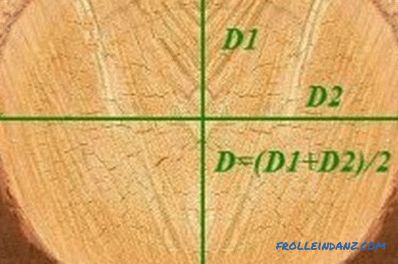Figure 1. Measurement of two perpendicular diameters in the beam section

If round wood characterized by the same length of logs and close diameter, then you can calculate the amount of 3 bars, reduce them to the average calculated value and multiply it by the amount of log material. In this case, the calculations are performed as follows:

1. Select 3 any bars.
2. Measure their diameter and length. For convenience of calculation, make measurements in meters.
3. Calculate the cubic space occupied by each of the rounds using the formula:

V = Pi * R 2 * L,

where Pi - the number equal to 3.14,

R is the radius equal to half the measured diameter,

L is the length of the beam.

1. The obtained values ​​of 3 cubic meters of round wood will be close to each other. They need to be added and the amount divided by 3. Thus, we obtain the value of the average volume of one log.
2. Next, multiply the average value obtained by the number of blanks in the machine or storage.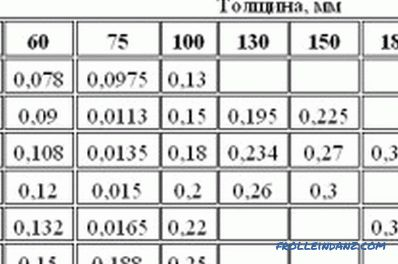Table for calculating the cubic capacity of logs and beams.

Since logs of similar diameter and standardized length are used for construction, this method is widely used to estimate the volume of construction timber.

If the form of a bar is slightly pressed down, its section in section will be not round, but slightly oval. How then to determine the value of the diameter? To do this, measure the size of 2 perpendicular diameters in the cross section of the beam: the largest and smallest. Further, the measured values ​​are summed and divided by 2. The resulting value will be the average diameter used in the calculations (Fig. 1).

## Calculation of the volume by tables

The calculation of the volume of round wood using tables consists of measuring the diameter and obtaining data from finished construction tables.

These directories contain structured information indicating the consumption of sawn timber of a given diameter for the construction of 1 m² wall. Such tables are the result of construction calculations and the established practice of determining the consumption of lumber. Therefore, they allow a fairly accurate estimate of the cubic capacity of the construction wood. A set of tables summarized in a single standardized document is called a GOST.

Most often, the tables are calculated for a rounded log, in which the top layer and bark are removed, the surface is aligned to the correct cylinder.

If you wish, you can use the data in the tables to determine the amount of un-pruned tree, making allowance for possible inaccuracies. Errors of the results will be associated with changes in the diameter of the cross section of the round timber over its length.

Independent calculation of the amount of roundwood allows you to verify the correctness of the price determination by the seller. It is economically feasible to check the team of contractors by conducting their own approximate calculations of the consumption of construction logs for the construction of the walls of the house.

•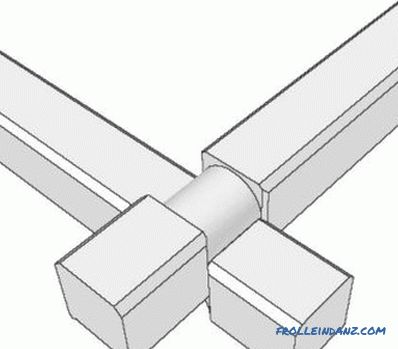How to determine how many cubes are in a cube?

Recommendations on how to correctly calculate how much a bar is in a cube. Calculation of the required amount of timber. The amount of material in one cube. Calculate total losses.

•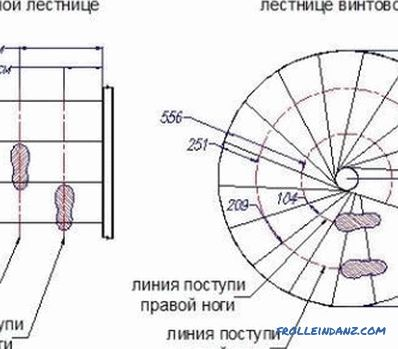How can I calculate the stairs?

Information on how to calculate the stairs in a private house. Some common rules for computing. The choice of the optimal parameters of staircases and individual steps.